# PSAT Math : Percentage

## Example Questions

### Example Question #51 : Percentage

The cost of a hat increases by 15% and then decreases by 35%. After the two price changes, the new price of the hat is what percent of the original?

85.3%

80%

74.75%

75%

88.91%

74.75%

Explanation:

The easiest way to do percentage changes is to keep them all in one equation. Therefore, we would say that an increase of 15% is the same as multiplying the original value by 1.15. Likewise, we would say that a discount by 35% is the same as multiplying the original by .65.

For our problem, let the hat cost X dollars originally. Therefore, after its increase, it costs 1.15X dollars. Now, we can consider this new price as the whole to which the discount will be applied. Therefore, a 35% reduction is (1.15X) * 0.65.

Simplifying, we get 0.7475, or 74.75%.

### Example Question #1 : How To Find The Percent Of Decrease

A dress is reduced in price by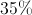, but it still doesn't sell, so the manager discounts it by another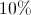. What is the total percentage discount?

None of the other answer choices are correct.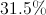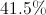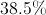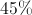Explanation:

For these type of questions, it is always best to pretend that we are beginning with a $100 item and to calculate from there.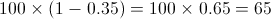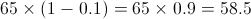If an item that is$100 is discounted by 35%, and then another 10%, the new price is 58.5%.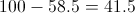The price difference (discount) is $41.5 for every$100, or: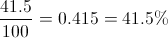The total discount is 41.5%.

### Example Question #1 : Percent Of Change

The population of Town A is 12,979 people in 1995.  The population, when measured again in 2005, is 22,752.  What was the change in population to the nearest whole percentage point?

75%

68%

43%

175%

57%

75%

Explanation:

Since we are looking for the change, we must take the

(Ending Point – Starting Point)/Starting Point * 100%

(22752 – 12979)/12979 * 100%

9773/12979 * 100%

0.753 * 100%

75%

### Example Question #1 : How To Find The Percent Of Increase

A factory produced 2500 units during the month of September.  In order to increase production by 12% in the month of October, the factory hired more workers.  How many units were produced in October?

3000

3600

3200

4000

2800

2800

Explanation:

This is a percentage increase problem.

Easiest approach :  2500 x 1.12 = 2800

In this way you are adding 12% to the original.

Using the formula, find 12% of 2500

12/100 = x/2500,

30000 = 100x

300 = x

Now add that to the original to find the new production:

2500 + 300 = 2800

### Example Question #1 : How To Find The Percent Of Increase

The radius of a given circle is increased by 20%.  What is the percent increase of the area of the circle.

20%

100%

144%

40%

44%

44%

Explanation:

If we plug-in a radius of 5, then a 20% increase would give us a new radius of 6 (which is 1.2 x 5).  The area of the new circle is π(6)2 = 36π, and the area of the original circle was π(5)2 = 25π .  The numerical increase (or difference) is 36π - 25π = 11π.  Next we have to divide this difference by the original area: 11π/25π = .44, which multiplied by 100 gives us a percent increase of 44%.  The percent increase = (the numerical increase between the new and original values)/(original value) x 100. The algebraic solution gives us the same answer.  If radius r of a certain circle is increased by 20%, then the new radius would be (1.2)r.  The area of the new circle would be 1.44 π r2 and the area of the original circle πr2.  The difference between the areas is .44 π r2, which divided by the original area, π r2, would give us a percent increase of .44 x 100 = 44%.

### Example Question #1 : How To Find The Percent Of Increase

Phoenicia is a grocery store that is expanding quickly.

In 2011 Phoenicia's total sales were $1,800,800. In 2012 their sales rose to$2,130,346.

By what percentage did the store increase its income from 2011 to 2012.

(Round answer to the nearest tenth.)

10.5%

18.3%

19.2%

21.0%

16.4%

18.3%

Explanation:

$1,800,800 divided by 100 equals 18,008 and$2,130,346 divided by 18,008 is 118.3

So we know that \$2,130,346 is 118.3% of the sales in the previous year. Hence sales increased by 18.3%.

### Example Question #1 : How To Find The Percent Of Increase

Cindy is running for student body president and is making circular pins for her campaign. She enlarges her campaign image to fit the entire surface of a circular pin. After the image is enlarged, its new diameter is 75 percent larger than the original. By approximately what percentage has the area of the image increased?

100%

225%

75%

200%

20%

200%

Explanation:

Pick any number to be the original diameter. 10 is easy to work with. If the diameter is 10, the radius is 5. The area of the original image is A = πr2, so the original area = 25π. Now we increase the diameter by 75%, so the new diameter is 17.5. The radius is then 8.75. The area of the enlarged image is approximately 77π. To find the percentage by which the area has increased, take the difference in areas divided by the original area.  (77π - 25π)/25π = 51π/25π = 51/25 = 2.04 or approximately 200%

### Example Question #1 : How To Find The Percent Of Increase

If the side of a square is doubled in length, what is the percentage increase in area?Explanation:

The area of a square is given by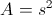, and if the side is doubled, the new area becomes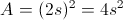.  The increase is a factor of 4, which is 400%.

### Example Question #51 : Percentage

A rectangle has a width of 6 and a length of 10. If both the width and length are increased by 2, what is the percent increase of the area of the rectangle?

37.5%

60%

50%

36%

160%

60%

Explanation:

The equation to use for percent increase or decrease is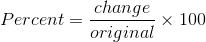to find the change in area, let's first calculate the area of the original. The formula for area of a rectangle is area=length x width. The original area, therefore, is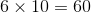The new rectangle has side lengths which are increased by 2, so the new lengths are 8 and 12. The area of the new rectangle, then is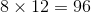.

The change in area is found by subtracting the the old area from the new area, so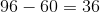When you input these numbers into the percent increase equation, you get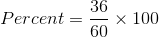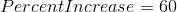### Example Question #1 : How To Find Percentage Equivalent To A Decimal

If 20% of x is equal to 160% of y, then y is what percent of x?

12.5

800

1.25

8

125

12.5

Explanation:

We are told that 20% of x is equal to 160% of y. We need to write expressions for 20% of x and 160% of y and set them equal to one another.

In order to find an expression for 20% of x, we can write 20% as a decimal and multiply it by x. Since 20% = 0.20, we can write 20% of x as 0.2x.

Similarly, we can write 160% as 1.60y.

Now, we set these expressions equal to one another.

0.2x = 1.60y.

Since the question asks us to find y as a percentage of x, we need to solve for y in terms of x. Let's divide both sides of the equation by 1.60.

0.125x = y.

Therfore, y is equal to 0.125x, which is the same as 12.5% of x, since 12.5% expressed as a decimal is 0.125.

The answer is 12.5.

### All PSAT Math Resources